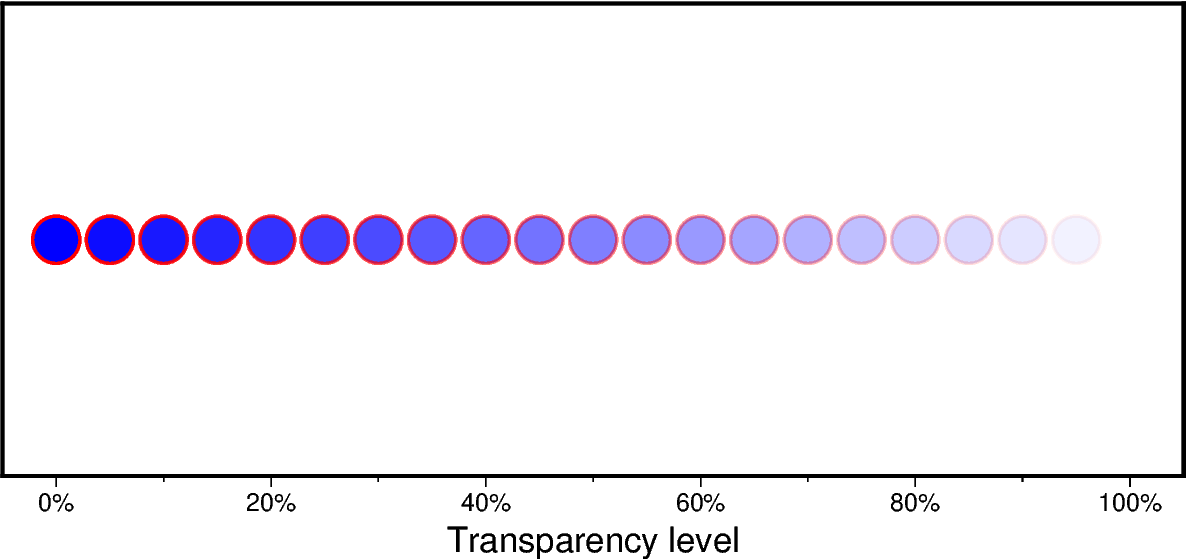# Points with varying transparency

Points can be plotted with different transparency levels by passing in an array argument to the `transparency` parameter of `pygmt.Figure.plot`.Out:

```<IPython.core.display.Image object>
```

```import numpy as np
import pygmt

# prepare the input x and y data
x = np.arange(0, 105, 5)
y = np.ones(x.size)
# transparency level in percentage from 0 (i.e., opaque) to 100
transparency = x

fig = pygmt.Figure()
fig.basemap(
region=[-5, 105, 0, 2],
frame=['xaf+l"Transparency level"+u%', "WSrt"],
projection="X15c/6c",
)
fig.plot(x=x, y=y, style="c0.6c", color="blue", pen="1p,red", transparency=transparency)
fig.show()
```

Total running time of the script: ( 0 minutes 1.284 seconds)

Gallery generated by Sphinx-Gallery